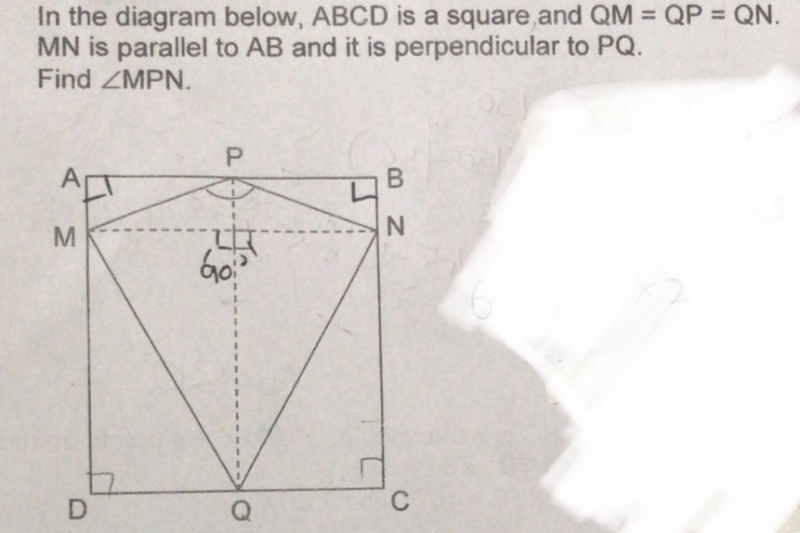QuestionTriangle MNQ is equilateral (QM = QN = QP = MN)

Angle MQN = 60 deg

Angle MQP = 30 deg

Angles QMP = MPQ (isosceles triangle because QM = QP)

Let angle QMP = QPM = QPN = QNP = angle X

2X + 30 = 180 (triangle QMP)

2X = 150

X = 75

Angle MPN = angle QPM + angle QPM = 150

0 Replies 0 Likes